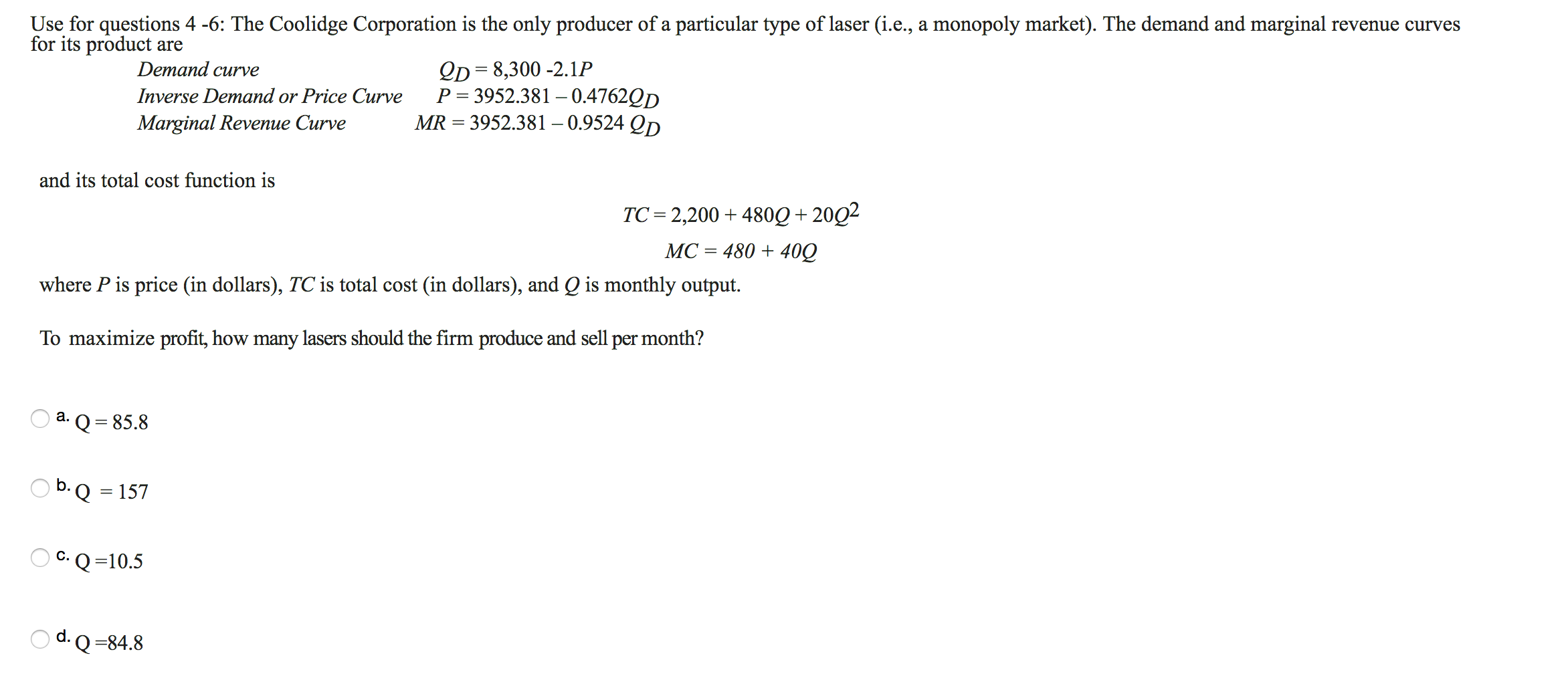Use for questions 4 -6: The Coolidge Corporation is the only producer of a particular type of laser (i.e., a monopoly market). The demand and marginalfor its product arerevenue curvesQD 8,300-2.1PP 3952.381 -0.4762QDMR 3952.381 -0.9524 QpDemand curveInverse Demand or Price CurveMarginal Revenue Curveand its total cost function isTC 2,200 480Q+2002МС %3 480 + 400where P is price (in dollars), TC is total cost (in dollars), and Q is monthly outputTo maximize profit, how many lasers should the firm produce and sell per month?а.Q 85.8b.Q 157C. Q =10.5d. Q84.8

Questionhelp_outlineImage TranscriptioncloseUse for questions 4 -6: The Coolidge Corporation is the only producer of a particular type of laser (i.e., a monopoly market). The demand and marginal for its product are revenue curves QD 8,300-2.1P P 3952.381 -0.4762QD MR 3952.381 -0.9524 Qp Demand curve Inverse Demand or Price Curve Marginal Revenue Curve and its total cost function is TC 2,200 480Q+2002 МС %3 480 + 400 where P is price (in dollars), TC is total cost (in dollars), and Q is monthly output To maximize profit, how many lasers should the firm produce and sell per month? а. Q 85.8 b.Q 157 C. Q =10.5 d. Q84.8 fullscreen
Step 1

Condition for profit maximization in monopoly is MR=MC

MR =3952.381 -0.9524 Qd

MC=480 + 40 Q

3...

Want to see the full answer?

See Solution

Want to see this answer and more?

Our solutions are written by experts, many with advanced degrees, and available 24/7

See Solution
Tagged in